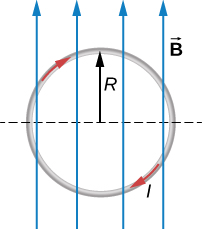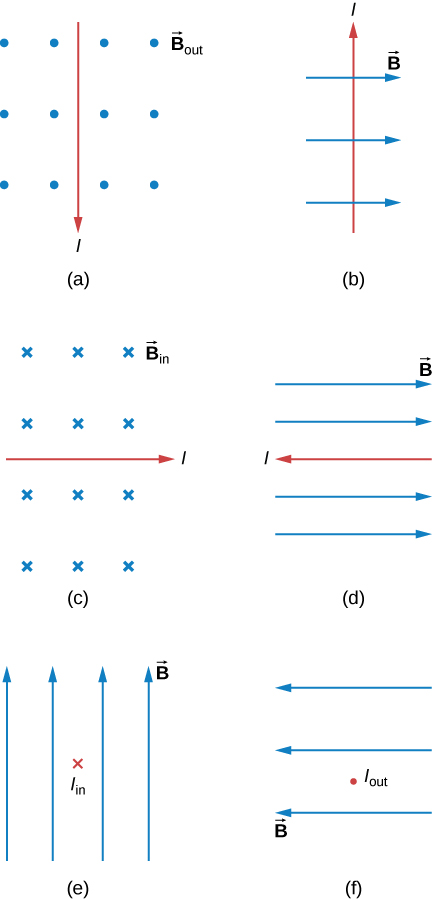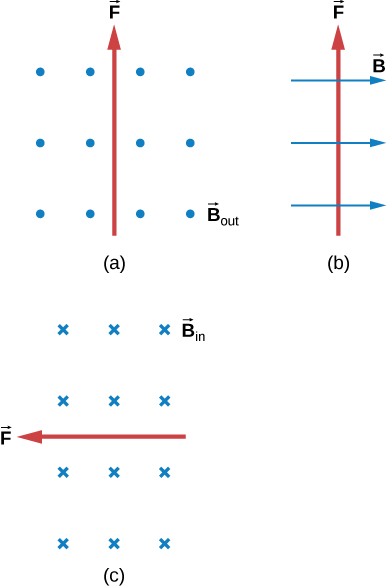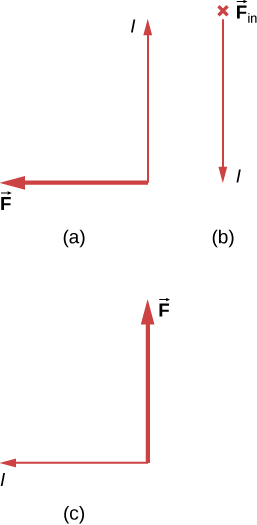# 11.4 Magnetic force on a current-carrying conductor  (Page 3/3)

 Page 3 / 3

## Solution

1. We start with the general formula for the magnetic force on a wire. We are looking for the force per unit length, so we divide by the length to bring it to the left-hand side. We also set $\text{sin}\phantom{\rule{0.1em}{0ex}}\theta =1.$ The solution therefore is
$\begin{array}{ccc}\hfill F& =\hfill & IlB\phantom{\rule{0.2em}{0ex}}\text{sin}\phantom{\rule{0.1em}{0ex}}\theta \hfill \\ \hfill \frac{F}{l}& =\hfill & \left(5.0\phantom{\rule{0.2em}{0ex}}\text{A}\right)\left(\text{0.30 T}\right)\hfill \\ \hfill \frac{F}{l}& =\hfill & 1.5\phantom{\rule{0.2em}{0ex}}\text{N/m.}\hfill \end{array}$

Directionality: Point your fingers in the positive y -direction and curl your fingers in the positive x -direction. Your thumb will point in the $\text{−}\stackrel{\to }{k}$ direction. Therefore, with directionality, the solution is
$\frac{\stackrel{\to }{F}}{l}=-1.5\stackrel{\to }{k}\phantom{\rule{0.2em}{0ex}}\text{N/m.}$
2. The current times length and the magnetic field are written in unit vector notation. Then, we take the cross product to find the force:
$\begin{array}{ccc}\hfill \stackrel{\to }{F}& =\hfill & I\stackrel{\to }{l}\phantom{\rule{0.2em}{0ex}}×\phantom{\rule{0.2em}{0ex}}\stackrel{\to }{B}=\left(5.0A\right)l\stackrel{^}{j}\phantom{\rule{0.2em}{0ex}}×\phantom{\rule{0.2em}{0ex}}\left(0.30T\text{cos}\left(30\text{°}\right)\stackrel{^}{i}+0.30T\phantom{\rule{0.1em}{0ex}}\text{sin}\left(30\text{°}\right)\stackrel{^}{j}\right)\hfill \\ \hfill \stackrel{\to }{F}\text{/}l& =\hfill & -1.30\stackrel{^}{k}\phantom{\rule{0.2em}{0ex}}\text{N/m.}\hfill \end{array}$

## Significance

This large magnetic field creates a significant force on a small length of wire. As the angle of the magnetic field becomes more closely aligned to the current in the wire, there is less of a force on it, as seen from comparing parts a and b.

Check Your Understanding A straight, flexible length of copper wire is immersed in a magnetic field that is directed into the page. (a) If the wire’s current runs in the + x -direction, which way will the wire bend? (b) Which way will the wire bend if the current runs in the – x -direction?

a. bends upward; b. bends downward

## Force on a circular wire

A circular current loop of radius R carrying a current I is placed in the xy -plane. A constant uniform magnetic field cuts through the loop parallel to the y -axis ( [link] ). Find the magnetic force on the upper half of the loop, the lower half of the loop, and the total force on the loop.A loop of wire carrying a current in a magnetic field.

## Strategy

The magnetic force on the upper loop should be written in terms of the differential force acting on each segment of the loop. If we integrate over each differential piece, we solve for the overall force on that section of the loop. The force on the lower loop is found in a similar manner, and the total force is the addition of these two forces.

## Solution

A differential force on an arbitrary piece of wire located on the upper ring is:

$dF=IB\phantom{\rule{0.1em}{0ex}}\text{sin}\phantom{\rule{0.1em}{0ex}}\theta \phantom{\rule{0.1em}{0ex}}dl.$

where $\theta$ is the angle between the magnetic field direction (+ y ) and the segment of wire. A differential segment is located at the same radius, so using an arc-length formula, we have:

$\begin{array}{ccc}\hfill dl& =\hfill & R\phantom{\rule{0.1em}{0ex}}d\theta \hfill \\ \hfill dF& =\hfill & IBR\phantom{\rule{0.1em}{0ex}}\text{sin}\phantom{\rule{0.1em}{0ex}}\theta \phantom{\rule{0.1em}{0ex}}d\theta .\hfill \end{array}$

In order to find the force on a segment, we integrate over the upper half of the circle, from 0 to $\pi .$ This results in:

$F=IBR{\underset{0}{\overset{\pi }{\int }}\text{sin}\phantom{\rule{0.1em}{0ex}}\theta \phantom{\rule{0.1em}{0ex}}d\theta =IBR\left(\text{−}\text{cos}\pi +\text{cos}0\right)}^{}=2IBR.$

The lower half of the loop is integrated from $\pi$ to zero, giving us:

$F=IBR{\underset{\pi }{\overset{0}{\int }}\text{sin}\phantom{\rule{0.1em}{0ex}}\theta \phantom{\rule{0.1em}{0ex}}d\theta =IBR\left(\text{−}\text{cos}0+\text{cos}\pi \right)}^{}=-2IBR.$

The net force is the sum of these forces, which is zero.

## Significance

The total force on any closed loop in a uniform magnetic field is zero. Even though each piece of the loop has a force acting on it, the net force on the system is zero. (Note that there is a net torque on the loop, which we consider in the next section.)

## Summary

• An electrical current produces a magnetic field around the wire.
• The directionality of the magnetic field produced is determined by the right hand rule-2, where your thumb points in the direction of the current and your fingers wrap around the wire in the direction of the magnetic field.
• The magnetic force on current-carrying conductors is given by $\stackrel{\to }{F}=I\stackrel{\to }{l}\phantom{\rule{0.2em}{0ex}}×\phantom{\rule{0.2em}{0ex}}\stackrel{\to }{B}$ where I is the current and l is the length of a wire in a uniform magnetic field B .

## Conceptual questions

Describe the error that results from accidently using your left rather than your right hand when determining the direction of a magnetic force.

Considering the magnetic force law, are the velocity and magnetic field always perpendicular? Are the force and velocity always perpendicular? What about the force and magnetic field?

Velocity and magnetic field can be set together in any direction. If there is a force, the velocity is perpendicular to it. The magnetic field is also perpendicular to the force if it exists.

Why can a nearby magnet distort a cathode ray tube television picture?

A magnetic field exerts a force on the moving electrons in a current carrying wire. What exerts the force on a wire?

A force on a wire is exerted by an external magnetic field created by a wire or another magnet.

There are regions where the magnetic field of earth is almost perpendicular to the surface of Earth. What difficulty does this cause in the use of a compass?

## Problems

What is the direction of the magnetic force on the current in each of the six cases?a. left; b. into the page; c. up; d. no force; e. right; f. down

What is the direction of a current that experiences the magnetic force shown in each of the three cases, assuming the current runs perpendicular to $\stackrel{\to }{B}$ ?What is the direction of the magnetic field that produces the magnetic force shown on the currents in each of the three cases, assuming $\stackrel{\to }{B}$ is perpendicular to I ?a. into the page; b. left; c. out of the page

(a) What is the force per meter on a lightning bolt at the equator that carries 20,000 A perpendicular to Earth’s $3.0\phantom{\rule{0.2em}{0ex}}×\phantom{\rule{0.2em}{0ex}}{10}^{-5}\text{T}$ field? (b) What is the direction of the force if the current is straight up and Earth’s field direction is due north, parallel to the ground?

(a) A dc power line for a light-rail system carries 1000 A at an angle of 30.0º to Earth’s $5.0\phantom{\rule{0.2em}{0ex}}×\phantom{\rule{0.2em}{0ex}}{10}^{-5}\text{T}$ field. What is the force on a 100-m section of this line? (b) Discuss practical concerns this presents, if any.

a. 2.50 N; b. This means that the light-rail power lines must be attached in order not to be moved by the force caused by Earth’s magnetic field.

A wire carrying a 30.0-A current passes between the poles of a strong magnet that is perpendicular to its field and experiences a 2.16-N force on the 4.00 cm of wire in the field. What is the average field strength?

#### Questions & Answers

what is the weight of the earth in space
As w=mg where m is mass and g is gravitational force... Now if we consider the earth is in gravitational pull of sun we have to use the value of "g" of sun, so we can find the weight of eaeth in sun with reference to sun...
Prince
g is not gravitacional forcé, is acceleration of gravity of earth and is assumed constante. the "sun g" can not be constant and you should use Newton gravity forcé. by the way its not the "weight" the physical quantity that matters, is the mass
Jorge
Yeah got it... Earth and moon have specific value of g... But in case of sun ☀ it is just a huge sphere of gas...
Prince
Thats why it can't have a constant value of g ....
Prince
not true. you must know Newton gravity Law . even a cloud of gas it has mass thats al matters. and the distsnce from the center of mass of the cloud and the center of the mass of the earth
Jorge
sun doesnt cause weight... it causes centripetal force....so it experiences weightlessness
ing
sun causes GRAVITATIONAL FORCE
Jorge
please why is the first law of thermodynamics greater than the second
define electric image.obtain expression for electric intensity at any point on earthed conducting infinite plane due to a point charge Q placed at a distance D from it.
explain the lack of symmetry in the field of the parallel capacitor
pls. explain the lack of symmetry in the field of the parallel capacitor
Phoebe
clean ur cars
ing
does your app come with video lessons?
What is vector
Vector is a quantity having a direction as well as magnitude
Damilare
tell me about charging and discharging of capacitors
a big and a small metal spheres are connected by a wire, which of this has the maximum electric potential on the surface.
3 capacitors 2nf,3nf,4nf are connected in parallel... what is the equivalent capacitance...and what is the potential difference across each capacitor if the EMF is 500v
equivalent capacitance is 9nf nd pd across each capacitor is 500v
santanu
four effect of heat on substances
why we can find a electric mirror image only in a infinite conducting....why not in finite conducting plate..?
because you can't fit the boundary conditions.
Jorge
what is the dimensions for VISCOUNSITY (U)
Branda
what is thermodynamics
the study of heat an other form of energy.
John
heat is internal kinetic energy of a body but it doesnt mean heat is energy contained in a body because heat means transfer of energy due to difference in temperature...and in thermo-dynamics we study cause, effect, application, laws, hypothesis and so on about above mentioned phenomenon in detail.
ing
It is abranch of physical chemistry which deals with the interconversion of all form of energy
Vishal
what is colamb,s law.?
it is a low studied the force between 2 charges F=q.q`\r.r
Mostafa
what is the formula of del in cylindrical, polar media
prove that the formula for the unknown resistor is Rx=R2 x R3 divided by R3,when Ig=0.ByBy Qqq QqqBy